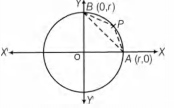# Q. Let AB be a chord of the circle $x^2 + y^2 = r^2$ subtending a right angle at the centre. Then, the locus of the centroid of the $\Delta PAB$ as P moves on the circle, is

IIT JEEIIT JEE 2001Conic Sections

Solution:

## Choosing OA as X-axis, A = (r,0), B = (0, r) and any point P on the circle is (r $cos\theta$, r $sin\theta$). If (x, y) is the centroid of $\Delta$ PAB, then 3x=r $cos\theta$ + r + 0 and 3y=r $sin \theta$ + 0 + r $\therefore$ $(3x - r)^2 + (3y - r)^2 = r^2$ Hence, locus of P is a circle.You must select option to get answer and solution

Integrals

Vector Algebra

KCET 2014

AIEEE 2002 Sets

## 2. For $r = 0, 1, ... , 10,$ if $A_r,B_r$ and $C_r$ denote respectively the coefficient of $x^r$ in the expansions of $(1 + x)^{10}, (1 + x)^{20}$ and $(1 + x)^{30}$. Then, $\displaystyle \sum A_r(B_{10}B_r-C_{10}A_r)$ is equal to

IIT JEE 2010 Binomial Theorem

## 3. Let $\omega \ne 1$ be a cube root of unity and S be the set of all non-singular matrices of the form $\begin {bmatrix} 1 & a & b \\ \omega & 1 & c \\ \omega^2 & \omega & 1 \end {bmatrix}$ where each of a , b and c is either $\omega \ or \ omega^2$ Then, the number of distinct matrices in the set S is

IIT JEE 2011 Determinants

## 4. Perpendicular are drawn from points on the line $\frac{x+2}{2}=\frac{y+1}{-1}= \frac{z}{3}$ to the plane $x+ y + z = 3$. The feet of perpendiculars lie on the line

JEE Advanced 2013 Introduction to Three Dimensional Geometry

## 5. The centre of the circle passing through the point (0, 1)and touching the curve $y = x^2 at (2,4)$ is

IIT JEE 1983 Conic Sections

## 6. A ray of light along $x+\sqrt 3 \, y=\sqrt 3$ gets reflected upon reaching X-axis, the equation of the reflected ray is

JEE Main 2013 Straight Lines

## 7. A ray of light along $x+\sqrt 3 \, y=\sqrt 3$ gets reflected upon reaching X-axis, the equation of the reflected ray is

JEE Main 2013 Straight Lines

## 8. A ray of light along $x+\sqrt 3 \, y=\sqrt 3$ gets reflected upon reaching X-axis, the equation of the reflected ray is

JEE Main 2013 Straight Lines